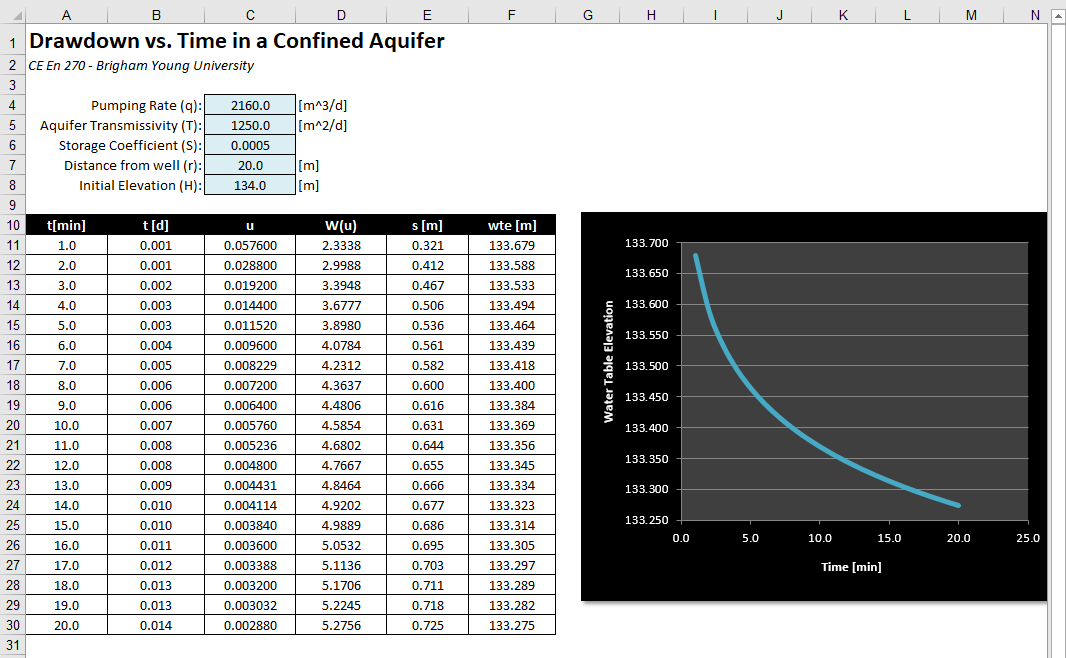# HW#20 - Well Function

When a well is placed in a confined aquifer and water is pumped from the well, a cone of depression occurs in the piezometric surface (water table) as follows: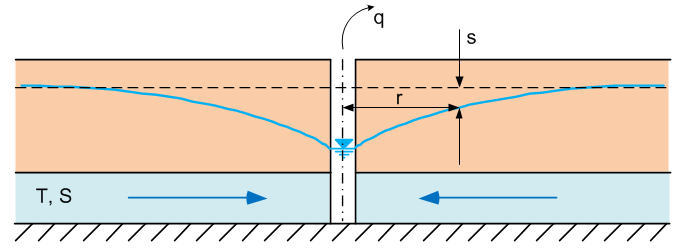The following equation can be used to the compute the drawdown of the water table as a result of pumping as a function of distance from the well (r) and time (t) since the pumping began: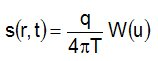The drawdown, s, is the difference between the current water table elevation and the initial horizontal water table elevation prior to turning on the well. The variable q is the pumping rate and the variable T is the aquifer transmissivity. The variable u is defined as: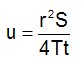where r is the distance from the well to the point in question, t is the time since pumping began, and S is the aquifer storage coefficient.  The last part of the drawdown equation, W(u), is the “well function” and corresponds to the integral: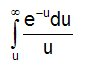The well function can be estimated from the series: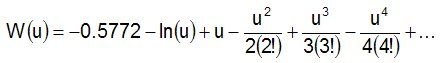where ln(u) is the natural log of u and n! is the factorial of n. Note that this equation can also be written as: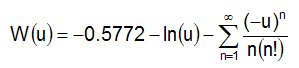The following spreadsheet is designed to calculate the drawdown vs. time at a point at a given distance, r, away from a well: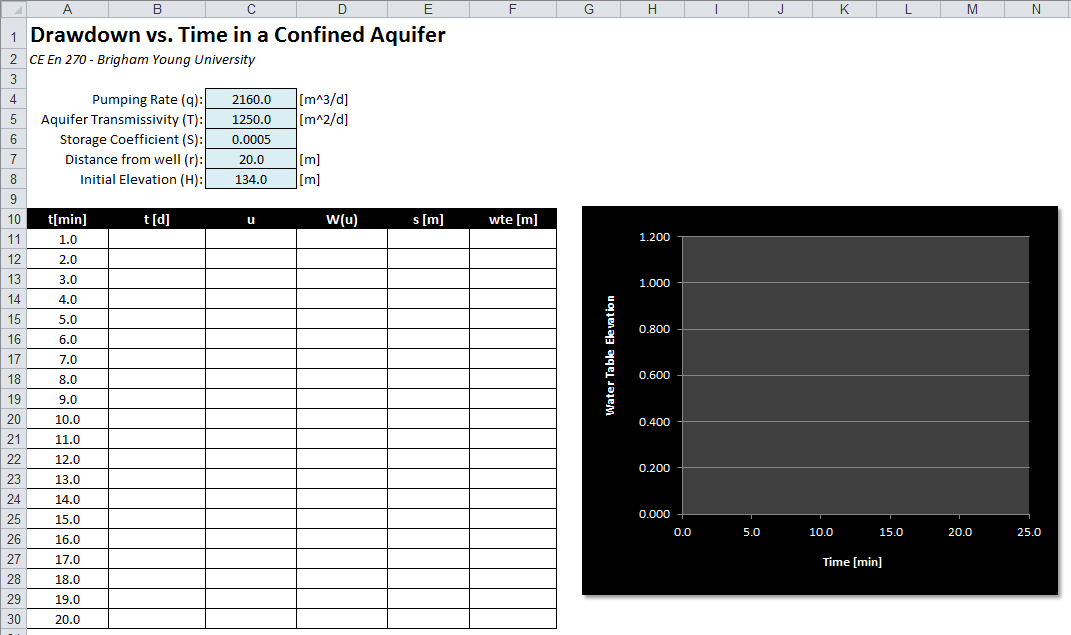Right click here to download a copy of this spreadsheet. Note that the blue cells at the top of the spreadsheet have all been named. In solving this problem, always reference these cells using the names. Note that the cell for r is named "rr".

a. A set of time values in minutes is listed in column A. Write a formula for column B that will convert the time values from minutes to days. This is necessary to ensure that we have consistent time units for all inputs used to calculate u.

b. Using the equation shown above, write a formula in column C for computing the variable u. Use the time value in days from column b and the other parameters from the top.

c. Using the equation shown above, write a custom function in VB for computing the well function W(u). Call your custom function wellf. The function should take a double representing u as an input argument and should return a double. To compute the well function you will need to write a loop that keeps adding terms to a summation until the next term is smaller than some small tolerance factor. Keep adding terms until the absolute value of the current term is less than 1e-12.

In order to compute the well function, you will first need to write a second VB function for computing the factorial of an integer. Recall that the factorial of a number is the number multiplied by each of the integers between 1 and the number - 1. For example 6! = 1*2*3*4*5*6. Your function should be called factorial and it should take a single integer, n, as an argument and it should return a double (it should be a whole number but we will use a double to avoid numerical overflow).

Your computed W(u) values should be somewhere in the range of 2-5 for this problem.

d. Use your custom well function in a formula to compute the well function values in Column D.

e. Using the drawdown equation shown above, write a formula in column E for computing the drawdown based on the well function values in column D.

f. Write a formula in column F for computing the water table elevation. The water table elevation is the initial water table elevation (H) minus the drawdown values in column E.

If you have entered the formulas correctly and defined the functions correctly, you should get the following set of numbers: